# Steiner system

(Redirected from Steiner triple system)

A pair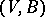, whereis a finite set ofelements andis a set of-subsets in(called blocks) such that every-subset fromis contained in exactly one block of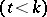. The numberis called the order of the Steiner system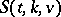. A Steiner system is a particular case of a block design and of a tactical configuration. A Steiner system foris a balanced incomplete block design (BIBD), and for,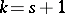it is a finite projective plane. A necessary condition for the existence of a Steiner systemis that the number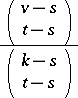is an integer for allsatisfying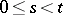. The sufficiency of this condition was proved for,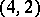,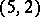,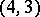(see , ).

In 1844 W. Woolhouse stated the existence problem for Steiner systems, and P. Kirkman solved it in 1847 for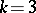(Steiner triple systems). In 1853 J. Steiner examined the case of.

Problems usually considered for Steiner systems are: 1) the determination of the maximum number of mutually non-isomorphic Steiner systems of a given order; 2) the existence of Steiner systems with a given group of automorphisms; 3) the imbedding of partial Steiner systems (not containing some-subsets of) in a finite Steiner system; 4) the existence of resolvable Steiner systems (withrepresentable as a union of partitions of); 5) the maximal packing (minimal covering) of a complete set of-subsets ofby disjoint(using Steiner systems).

The majority of results on Steiner systems are obtained for small values ofand(see ).

How to Cite This Entry:
Steiner triple system. Encyclopedia of Mathematics. URL: http://encyclopediaofmath.org/index.php?title=Steiner_triple_system&oldid=42968